# 1st PUC Physics Question Bank Chapter 9 Mechanical Properties of Solids

## Karnataka 1st PUC Physics Question Bank Chapter 9 Mechanical Properties of Solids

### 1st PUC Physics Mechanical Properties of Solids Textbook Questions and Answers

Question 1.
A steel wire of length 4.7 m and cross-sectional 3.0 × 10-5 m2 stretches by the same amount as a copper wire of length 3.5 m and cross-sectional area of 4.0 × 10-5 m2 under a given load. What is the ratio of the Young’s modulus of steel to that of copper?
Let the Young’s Modulus of steel and copper be Ys and Yc respectively.
Length of steel wire, Ls = 4.7 m
Length of copper wire, Lc = 3.5 m
Area of cross-section of steel wire,
As= 3 × 10-5 m2
Area of cross-section of copper wire,
Ac = 4 × 10-5 m2
Since change in lengths are equal.
∴ ∆Ls = ∆Lc = ∆ L.
As the load is same, Fs = Fc = F
We have, Y = $$\frac{F}{A} \times \frac{L}{\Delta L}$$
$$\therefore \frac{Y_{s}}{Y_{c}}=\frac{L_{s} A_{c}}{A_{s} L_{c}}=\frac{4 \times 4.7 \times 10^{-5}}{3 \times 3.5 \times 10^{-5}}$$ = 1.79
The ratio of Young’s Modulus of steel to that of copper is ≃ 1.8 :1.

Question 2.
Figure shows the strain – stress curve for a given material. What are
1. Young’s modulus and
2. approximate yield strength for this material?1. F from the given graph,
For a stress of 150 × 106 Nm-2
Strain = 0.002
∴ Young’s modulus (Y) = $$\frac{\text { stress }}{\text { strain }}$$
$$=\frac{150 \times 10^{6} \mathrm{Nm}^{-2}}{0.002}$$ = 7.5 × 1010 Nm-2

2. 300 × 106 Nm-2 is the maximum stress that the material can sustain without crossing the elastic limit. Thus, the yield strength of the material is 3 × 108 Nm-2 or 300 × 106 Nm-2.

Question 3.
The stress-strain graphs for materials A and B are shown in Figure.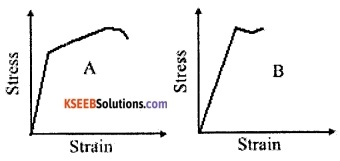The graphs are drawn to the same scale.

1. Which of the materials has the greater Young’s modulus?
2. Which of the two is the stronger material?

1. Material A has greater Young’s modulus as the slope of the graph of material A is greater than material B.
Young’s Modulus = Y = Slope = $$\frac{\text { stress }}{\text { strain }}$$
∴ Material A has greater Young’s Modulus.
2. From the graph it can be approximated that the stress at the fracture point for material A is higher than material B, thus material A is stronger.Question 4.
Read the following two statements below carefully and state, with reasons, if it is true or false.
1. The Young’s modulus of rubber is greater than that of steel;
2. The stretching of a coil is determined by its shear modulus.
1. The Young’s modulus of a -material defined as,
Y = $$\frac{\text { stress }}{\text { strain }}$$
Since, rubber stretches more than steel ∆ L for rubber is greater than that of steel, therefore ( $$\frac{\Delta L}{L}$$ 0f rubber) > ($$\frac{\Delta L}{L}$$ of steel) Since, Y $$\alpha \frac{1}{\text { strain }}$$ and, strain = $$\frac{\Delta L}{L}$$
Since the strain of rubber is greater, its Youngs modulus is less. Hence false,

2. The Stretching of a coil depends on the shear modulus of the material because for the coil to be stretched, a shear force has to applied to change the shape of the coil. Hence the statement is true.

Question 5.
Two wires of diameter 0.25 cm. one made of steel and the other made of brass are loaded as shown in Fig.9.13. The unloaded length of steel wire is 1.5 m and that of brass wire is 1.0 m. Compute the elongations of steel and the brass wires.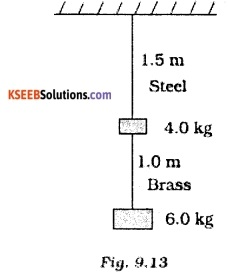given, d = 0.25 cm
r = $$\frac{\mathrm{d}}{2}$$ = 0.125 cm.
As = AB = π × (0.125 × 10-2)2 m2
= 4.91 × 10-6 m2
As = AB because the diameters are same.
Fs = (4 × g + 6 × g) N
= (4 × 9.8 + 6 × 9.8) N
= 10 × 9.8 N =98 N
FB = (6 × 9.8) N = 58.8 N
∆Ls = ?
∆LB = ?
Ls = 1.5 m
LB =1.0m
Ys = 2 × 1011 N m-2 and
YB = 0.91 × 1011 Nm-2
∆Ls = $$\frac{F_{s} \cdot L_{s}}{A_{s} \cdot Y_{s}}=\frac{98 \times 1.5}{4.91 \times 10^{-6} \times 2 \times 10^{11}} \mathrm{m}$$
= 1.49 × 10-4m = 0.149 mm
To find the elongation of Brass,
∆LB = $$\frac{F_{B} L_{B}}{A_{B} Y_{B}}=\frac{(58.8)(1)}{\left(4.91 \times 10^{-6}\right)\left(0.91 \times 10^{11}\right)} \mathrm{m}$$
= 1.32 × 10-4m = 0.132 mm
The elongation of steel wire is 0.149 mm.
The elongation of Brass wire is 0.132 mm.

Question 6.
The edge of an aluminium cube is 10 cm long. One face of the cube is firmly fixed to a vertical wall. A mass of 100 kg is then attached to the opposite face of the cube. The shear modulus of aluminium is 25 GPa. What is the vertical deflection of this face?
Edge length, L = 10 cm = 0.1 m
Weight attached = 100 kg
F = (100 × 9.8) N = 980 N
A = L2 = 0.1 × 0.1 m2 = 0.01 m2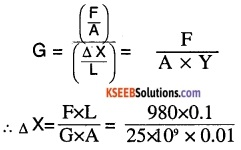= 3.92 × 10-7 m
∴ the deflection is 3.92 × 10-7 m

Question 7.
Four identical hollow cylindrical columns of mild steel support a big structure of mass of 50,000 kg. The inner and outer radii of each column are 30 and 60 cm respectively. Assuming the load distribution to be uniform, calculate the compressional strain of each column.
Mass of big structure = 50,000 kg
Net force = (5 × 104 × 9.8) N
= 4.9 × 105 N = FB
Since it is supported by four cylinders the weight is equally distributed.
Force (F) on each cylinder is
F = $$\frac{F_{B}}{4}$$ = 122500 N
Young’s Modulus = Y = = $$\frac{\text { stress }}{\text { strain }}=\frac{F \times L}{A \times \Delta L}$$
∴ Compressional strain of each column is given by, $$\frac{\Delta L}{L}=\frac{F}{A \times Y}$$
Here, F = 122500 N
A = π(0.6)2 – π(0.3)2 = π(0.36 – 0.09)
= 0.27 π = 8.48 × 10-1 m2
Y = 2 × 1011 N m-2 (Standard Values)
∴ Strain = $$\frac{122500}{(0.27 \pi)\left(2 \times 10^{11}\right)}$$ = 7.22 × 10-7
Thus the compressional strain per cylinder is 7.22 × 10-7Question 8.
A piece of copper having a rectangular cross-section of 15.2 mm × 19.1 mm is pulled in tension with 44,500 N force, producing only elastic deformation. Calculate the resulting strain?
F = 44,500 N
Strain =?
Length = 19.1 mm, Breath = 15.2 mm
Area = A = 19.1 × 15.2 × 10-6m2
= 2.98 × 1 O-4 m2
G = 42 × 109 Nm-2
We have, G = $$\frac{F}{A \times \text { strain }}$$
∴ Strain = $$\frac{F}{G \times A}$$
$$=\frac{44,500}{2.98 \times 10^{-4} \times 42 \times 10^{9}}$$
= 3.65 × 10-3

Question 9.
A steel cable with a radius of 1.5 cm supports a chair lift at a ski area. If the maximum stress does not exceed 108 Nm-2, What is the maximum load the cable can support?
Maximum load = maximum stress x area of cross-section
= 108 n r2 =108 X (22/7) x (1.5 x 10-2)2 = 7.07 x 104 N

Question 10.
A rigid bar of mass 15 kg is supported symmetrically by three wires each 2.0 m long. Those at each end are of copper and the middle one is of Iron. Determine the ratios of their diameters if each is to have the same tension.
Since the rigid bar is supported symmetrically by three wires each of length 2 m, the extension is same in all the three wires. Thus strain is same.
$$Y=\frac{F}{\pi r^{2} \times \text { strain }}$$ = $$\frac{4 \mathrm{F}}{\pi \mathrm{d}^{2} \times \text { strain }}$$
∴ Y $$\alpha \frac{1}{\mathrm{d}^{2}}$$
Yi = Young’s modulus of Iron
di = diameter of iron wire.
Yc = Young’s modulus of copper
dc = diameter of copper wire.
Yi = 190 × 109 N m-2
Yc = 110 × 109 N m-2
$$\frac{d_{c}}{d_{i}}=\sqrt{\frac{Y_{i}}{Y_{c}}}=\sqrt{\frac{19}{11}}=1.31: 1$$

Question 11.
A 14.5 kg mass, fastened to the end of a steel wire of unstretched length 1.0 m, is whirled in a vertical circle with an angular velocity of 2 rev/s at the bottom of the circle. The cross-sectional area of the wire is 0.065 cm2. Calculate the elongation of the wire when the mass is at the lowest point of its path.
L = 1 m
W = 2 revolutions/s
A = 0.065 cm2 = 6.5 x 10-6 m2
F = mg + mlw2
= 14.5 × 9.8 +14.5 × 1 × (2)2
= 200.1 N
∆L = $$\frac{F l}{A Y}$$                [∵ Y = $$\frac{\mathrm{FI}}{\mathrm{A} \Delta \mathrm{I}}$$]
Young’s modulus of steel is 2 × 1011 Nm-2
∆L = $$\frac{200.1 \times 1}{6.5 \times 10^{-6} \times 2 \times 10^{11}} \mathrm{m}$$ = 1.539 × 10-4m

Question 12.
Compute the bulk modulus of water from the following data: Initial Volume = 100.0 litre. Pressure increase = 100.0 atm (1 atm = 1.013 × 105 Pa), Final volume = 100.5 litre. Compare the bulk modulus of water with that of air (at constant temperature). Explain in simple terms why the ratio is so large.
VF = final volume = 100.5 I
= 100.5 × 10-3 m3
Vi = initial volume = 100 × 10-3 m3
∆V = VF – Vi =0.5 × 10-3 m3
= 5 × 10-4 m3
∆ P = 100 atm = 100 × 1.013 × 105 Pa= 2.03 × 109 Pa
Bulk modulus of air = 1 × 105 Pa
$$\frac{\text { Bulk modulus of water }}{\text { Bulk modulus of air }}=\frac{2.026 \times 10^{9}}{1 \times 10^{5}}$$
= 2.026 × 104
Since air is highly compressible compared to water, the ratio is so high.

Question 13.
What is the density of water at a depth where pressure is 80.0 atm, given that its density at the surface is 1.03 × 103 kg m-3?
Density of water at surface
= $$\rho_{1}$$ = 1.03 × 103 kgm-3
density of water at depth h = $$\rho_{2}$$
Pressure at depth h = 80 atm
= 80 × 1.01 × 105 Pa.
V1 = Volume of water of mass m at surface.
V2 = Volume of water at depth h.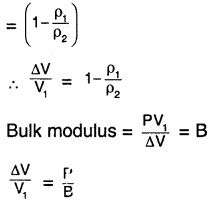$$\frac{1}{\mathrm{B}}$$ Compressibility of water
= 45.8 × 10-11
∴ $$\frac{\Delta V}{V_{1}}$$ =80 × 1.013 × 105 × 45.8 × 10-11
= 3.71 × 10-3
Since,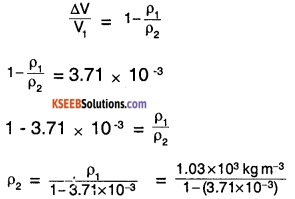= 1.034 × 103 kg m-3

Question 14.
Compute the fractional change in volume of a glass slab, when subjected to hydraulic pressure of 10 atm.
Hydraulic pressure exerted = 10 atm
= 10 × 1.013 × 105 Pa
B = $$\frac{P \cdot V}{\Delta V}$$
$$\frac{\Delta V}{V}$$ is the fractional change in volume
$$\frac{\Delta V}{V}=\frac{P}{B}=\frac{10 \times 1.013 \times 10^{5}}{37 \times 10^{9}}$$
= 2.73 × 10-5
∴ The fractional change in Volume is 2.73 × 10-5.

Question 15.
Determine the volume contraction of a solid copper cube, 10 cm on an edge, when subjected to a hydraulic pressure of 7.0 × 106 Pa.
P = 7 × 106 Pa
I = 10 cm
V = I3= 10-3 m3
B = 140 × 109
B = $$\frac{P \times V}{\Delta V}$$
∆V = $$\frac{P}{B} \times V=\frac{7 \times 10^{6}}{140 \times 10^{9}} \times 10^{-3} \mathrm{m}^{3}$$
= 5 × 10-8 m3
= 0.05 cm3
∴ The contraction is 0.05 cm3Question 16.
How much should the pressure on a litre of water be changed to compress it by 0.10%
P =?
V = 1L ,
B = 2.2 × 109Nm-2
$$\frac{\Delta v}{V}$$ × 100 = 0.1
⇒ $$\frac{\Delta v}{V}$$ = $$\frac{0.1}{100 \times 1}$$ = 10-3
Since, B = $$\frac{\mathrm{PV}}{\Delta \mathrm{V}}$$
P = B $$\frac{\Delta v}{V}$$
= 2.2 × 109 Pa × 10-3
= 2.2 × 106 Pa.
∴ The pressure is required is 2.2 × 106 Pa.

1st PUC Physics Mechanical Properties of Solids Additional Exercises Questions and Answers

Question 1.
Anvils made of single crystals of diamond, with the shape as shown in Fig. are used to investigate behaviour of materials under very high pressures. Flat faces at the narrow end of the anvil have a diameter of 0.50 mm, and the wide ends are subjected to a compressional force of 50,000 N. What is the pressure at the tip of the anvil?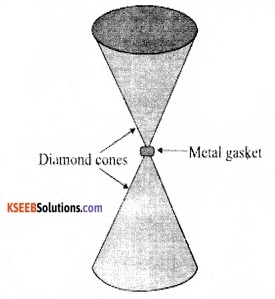Diameter at narrow end
= d =0.5 mm
Compressional Force = F = 50,000 N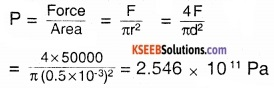∴ The pressure is 2.546 × 1011 Pa

Question 2.
A rod of length 1.05 m having negligible mass is supported at its ends by two wires of steel (wire A) and aluminium (wire B) of equal lengths as shown in Fig.9.15. The cross-sectional areas of wires A and B are 1.0 mm2 and 2.0 mm2 respectively. At what point along the rod should a mass m be suspended in order to produce.

1. equal stresses.
2. equal in both steel and aluminium wires.Let the initial lengths of the 2 wires be ‘L’
Given that the cross-sectional area of A
= Aa = 1 mm2 = 10-6
Cross-sectional area of
B = Ab = 2 mm2 = 2 × 10-6m2
Length of rod = 1.05 m.
Young’s modulus of wire A
= Ysteel = 2 × 1011 N/m2
Young’s modulus of wire B
YAl = 7 × 1010 N/m2
Let the mass ‘m’ be suspended at a distance ‘x’ from the end with wire A attached.

1. For equal stress on the two wires :
i.e., stress A = stress Bor Fb = 2 Fa …..(1)
where, Fa is force exerted on steel wire and Fσ is force exerted on aluminium wire. Since the total torque at the point when mass is suspended is zero, we can write
Fa × (x) = Fb × (1.05 – x)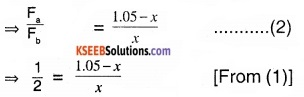⇒ 3x = 2 × 1.05 or x = 0.7 m
So, for equal stress on the wires the mass should be suspended at a distance 0.7m, from wire A.

2. For equal strain:
We know that, Strain = $$\frac{\text { Stress }}{Y}=\frac{F}{\text { AY }}$$
we require (strain)a = (strain)b$$\frac{F_{a}}{F_{b}}$$ = $$\frac{10}{7}$$
……………(3) Since the torque is ‘0’ at the point of suspension equation (2) is valid.
⇒ $$\frac{F_{a}}{F_{b}}$$ = $$\frac{10}{7}$$ = $$\frac{1.05-x}{x}$$
⇒ 17 x = 7 × (1.05)
x = 0.432 m
So, for equal strain on the wires the mass should be suspended at a distance 0.432 m from wire A.

Question 3.
A mild steel wire of length 1.0 m and cross-sectional area 0.50 × 10-2cm2 is stretched, well within its elastic limit, horizontally between two pillars. A mass of 100 g is suspended from the mid-point of the wire. Calculate the depression at the mid-point.
Given that length of the wire = 1.0 m
Area of cross-section = 0.50 × 10-2cm2
= 5.0 × 10-7m2
Mass suspended =100g
= 10-1 Kg
Ysteel = 2 × 1011 Pa
The mass causes the steel wire to bend as shown.
So new length of wire = AX + XB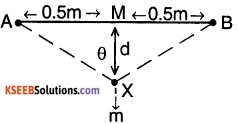Change in length is, ∆l = (AX + XB) – AB
Now, AX = $$\sqrt{\mathrm{AM}^{2}+\mathrm{MX}^{2}}$$ = XB
[ Pythogorus Theorem][Expanding and neglecting higher-order terms]
The tension in the steel wire is,
T cos θ + T cos θ = mg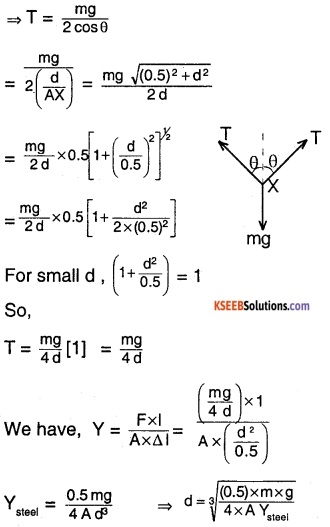$$\sqrt{\frac{0.5 \times 0.1 \times 9.8}{4 \times 2 \times 10^{11} \times 5 \times 10^{-7}}}$$ = 0.0106 m
So, the depression at the centre is 0.0106 m or 1.06 cm.

Question 4.
Two strips of metal are riveted together at their ends by four rivets, each of diameter 6.0 mm. What is the maximum tension that can be exerted by the riveted strip if the shearing stress on the rivet is not to exceed 6.9 × 107 Pa? Assume that each rivet is to carry one-quarter of the load.
Given diameter of metal strips
= 6.0 × 10-3 m
⇒ Area = $$\pi\left(\frac{\mathrm{d}}{2}\right)^{2}=\frac{\pi \times 36 \times 10^{-6} \mathrm{m}^{2}}{4}$$
= 2.827 × 10-5m2
For the maximum stress of 6.9 × 107 Pa
the maximum force is given by
Max Force = $$\frac{\text { Max Force }}{\text { Area }}$$
⇒ Max force = Max Stress × Area
= 6.9 × 107 × 2.827 × 10-5
= 1950.63 N
Since there are 4 rivets and each rivet carries 1/4th of the load,
Max tension that can be exerted by the rivet strip is 4 × 1.95063 KN
= 7.80252 KN.Question 5.
The Marina trench is located in the Pacific Ocean, and at one place it is nearly eleven km beneath the surface of water. The water pressure at the bottom of the trench Is about 1.1 × 108 Pa. A steel ball of Initial volume 0.32 m3 is dropped into the ocean and falls to the bottom of the trench. What is the change in the volume of the ball when it reaches to the bottom?
Pressure at bottom ’of the trench = ρgh = 1.1 × 108 Pa
Initial volume of the ball = 0.32 m3
Bulk modulus of steel = 1.6 × 1011 Pa
Let ∆v the change in volume of Ball when it falls down to the bottom.
We know that, B = $$\frac{P}{(\Delta V / V)}$$
⇒ ∆v = $$\frac{P V}{B}$$
$$=\frac{1.1 \times 10^{8} \times 0.32}{1.6 \times 10^{11}}$$ = 2.2 × 10-4 m3
Therefore, change in volume of the ball on reaching the bottom of the trench is 2.2 × 10-4 m3 or 220 cm3

### 1st PUC Physics Mechanical Properties of Solids One Mark Questions and Answers

Question 1.
What is elasticity?
The property of the body to restore the original shape or size on removal of the external force is called elasticity.

Question 2.
Define stress.
Stress is defined as the deforming force acting on a unit area of the body.

Question 3.
What is the S.l unit of stress?
Nm-2

Question 4.
Define strain.
Strain is the ratio of change in dimension of a body due to stress to the original dimension.Question 5.
Define Shear Strain.
The angular tilt between different layers of a body due to tangential force (Shear Force) is called Shear Strain.

Question 6.
Define Modulus of elasticity.
Modulus of elasticity is defined as the ratio of stress to strain.

Question 7.
State Hooke’s law.
Within elastic limit, stress is directly proportional to strain.

Question 8.
Mention the expression for Young’s modulus in the case of a stretched string.
Young’s modulus in case of a stretched string is Y = $$\frac{\mathrm{FL}}{\pi r^{2}(\Delta \mathrm{L})}$$
F – Force acting on the wire,
L – Initial length of the wire
∆L – change in length of the wire,
r – radius of the wire.

Question 9.
Which is more elastic, rubber or iron?
Iron.

Question 10.
What are anisotropic materials?
The materials which have different physical properties along different directions are called anisotropic materials.

Question 11.
Name 2 main groups of solids.
Amorphous and Crystalline.Question 12.
What are glass solids?
Glassy solids are amorphous solids.

Question 13.
What is the Bulk modulus of an incompressible object?
Since its incompressible strain is 0 and Bulk modulus is infinity.

Question 14.
Show graphically stress v/s strain curve for a Brittle material.Question 15.
If the length of the wire Is made 2 times, what Is the effect on the Increase in length under same load?
∆l ∝ l. So, increase in length is also doubled.

### 1st PUC Physics Mechanical Properties of Solids Two Marks Questions and Answers

Question 1.
If a wire is replaced by another similar wire but of half the diameter. What is the effect on

1. Increase in the length for a given load,
2. Max load It can sustain.

We know that, $$\frac{\Delta L}{L}=\frac{F}{A Y}$$
1.The increase in the length (∆L)is 4 times the original wire.

2.  We know that, F = F=A $$\left(\frac{\mathrm{Y} \Delta \mathrm{L}}{\mathrm{L}}\right)$$ Thus F ∝ A and hence maximum load it can sustain becomes 1/4th of the original wire.

Question 2.
What is a perfectly elastic and inelastic body?
If a body completely regains its natural shape or size after the removal of external forces, it is called a perfectly elastic body.
Eg: rubber.
If the body does not regain its original size or shape it is called inelastic body or plastic body.

Question 3.
Identical springs made of steel and aluminium are equally stretched. On which more work will have to be done? Why?
Work done for steel spring is more because steel is more elastic than aluminium, (alternatively: Spring constant of steel is more than that of aluminium).Question 4.
Why does a cycle tube burst in summer?
In summer as the temperature increases, the pressure increases inside the tube This pressure would be enough to cross the breaking point of the tyre making it to burst.

Question 5.
When the pressure on a sphere increases by 50 atm then its volume decreases by 0.02%. Find Bulk modulus.
P = 50 atm
P = 50 atm ≅ 50 × 1.01 × 105
$$\frac{\Delta V}{V}=\frac{0.02}{100}=2 \times 10^{-4}$$
Bulk modulus= $$\frac{\mathrm{P}}{(\Delta \mathrm{V} / \mathrm{V})}=\frac{5.05 \times 10^{6}}{2 \times 10^{-4}}$$
= 2.525 × 1010N/m2

Question 6.
Which is more elastic, rubber or Steel? Why?
For a given force, rubber extends more than steel, i.e., Steel is more elastic than rubber. This is because for the same amount of extension. Steel requires more force. For the same force ∆l ∝ Y, and F ∝ Y, for same extension Ysteel > Yrubber.

Question 7.
What is elastic Limit?
Elastic limit is the value of applied force beyond which the material (body) does not come back to its original shape when the applied force is reduced.

Question 8.
Plot load v/s extension curve for a substance on the graph show,

1. Yield point
2. Breaking point
3. Elastic limit
4. Crushing point.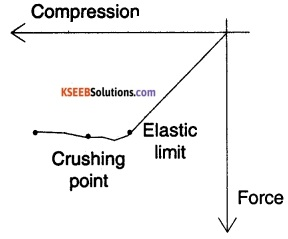Question 9.
The Young ‘s modulus of a wire of length L and radius ‘r’ is Y. If length is reduced to L/3 and Radius r/4. What will be Its ratio of extension?
Young’s modulus is given by
Y = $$\frac{F}{A} \frac{l}{\Delta}$$ ⇒ ∆l = $$\frac{F}{A} \frac{l}{Y}$$
So, ∆l ∝ l and ∆l ∝ $$\frac{1}{A}$$ and A ∝ r2Question 10.
The Young’s modulus of a material is Y. It has an area A and length ‘L’. Now if a is made $$\frac{A}{16}$$ and length L/2. What is the new Young’s modulus?
Even though the value of Young’s modulus is given by, Y = $$\frac{F}{A} \cdot \frac{l}{\Delta l}$$. It is only material dependent. It is constant for the given wire irrespective of its dimension. So, the new value of Young’s modulus is Y.Question 11.
The length of a metal is L1 when a force is F1 is applied on it and its length is L2 when applied force is F2. Find the original length of wire.
We know that Y = $$\frac{\mathrm{F} l}{\mathrm{A} \Delta l}$$
Let original length be L. Then,
L1 = L + ∆l1
L2 = L + ∆l2
Since, Y is a constant,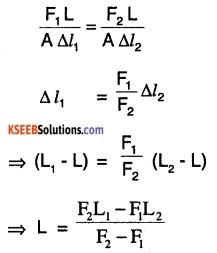Question 12.
Explain the terms Young’s modulus and elastic fatigue.
Young’s modulus is defined as the ratio of longitudinal stress to longitudinal strain.
Y = $$\frac{(F / A)}{(\Delta l / l)}$$
Elastic Fatigue is the time (delay) required for a material to regain its original position on removing the deforming force.

### 1st PUC Physics Mechanical Properties of Solids Three Marks Questions and Answers

Question 1.
When a wire is stretched by a certain force its elongation is ‘a’. If the second wire having double-radius and 4-time length. Find its elongation.= 4 × 4 a
∆l2 = 16 a.

Question 2.
Define stress and strain and derive their units. Write one limitation of Hooke’s Law.
Stress is the deforming force experience by the body per unit area.
Stress = $$\frac{F}{A}$$
Units = $$\frac{(N)}{\left(m^{2}\right)}$$ = Nm-2
Strain is the change in the considered quantity (l,v, A, θ…..) per unit of its original value.
Strain = $$\frac{\Delta x}{x}$$
It is unitless.
Hooke’s Law is valid only for small deformation i.e., in its limit.

### 1st PUC Physics Mechanical Properties of Solids Five Marks Questions and Answers

Question 1.
The Stress-Strain graph of a metal wire is shown in figure up to pint E. The wire returns to its original state 0 along EPO when it is gradually unloaded point B corresponds to the fracture of wire.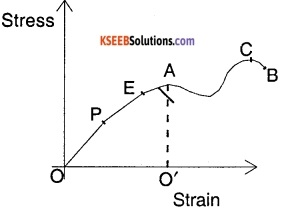1. Up to what point of the curve Hooke’s Law is obeyed?
2. Which point on the curve corresponds to yield point or elastic limit.
3. Indicate the Elastic region and plastic region.
4. Describe what happens when the wire Is loaded up to the stress corresponding to point A and then unloaded gradually. In particular to the dotted line.
5. What is peculiar about portion of curve from C to B? Upto what stress can be applied without causing fracture?

1. Since the graph is linear till point E. Hooke’s Law is obeyed till point E.
2. The point E corresponds to the elastic limit because till there the body returns to its original state along EPO.
3. Elastic – O to E; Plastic – E to B
4. Up to point E an almost linear increase in strain with stress. After point E, if it is loaded till A, the strain increases faster for some increase in stress. But on unloading the body returns along line AO to a permanent strain (change in shape) of O’.
5. In the curve C to B, the body undergoes a strain even if it is being unloaded and then fractures. To present fracture the value of stress has to be just less than that of C.

Question 2.
Explain different types of strain.
There are 3 types of strain.
1. Longitudinal strain :
It is defined as the ratio of change in length produced (dl) to the original length (L) of a thin rod.
Longitudinal strain = $$\frac{d l}{L}$$

2. Shearing strain :
It is defined as the ratio of displacement of a surface on which stress is acting to the height of the surface.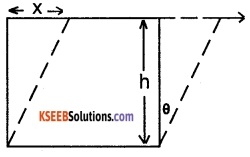Shearing strain θ = $$\frac{x}{h}$$
$$=\frac{\text { displacement }}{\text { heightof the surface }}$$

3. Bulk strain :
It is defined as the ratio of change in volume (dV) to the original volume (V).
Bulk strain = $$\frac{\mathrm{d} \mathrm{v}}{\mathrm{v}}$$.Question 3.
Explain the different types of elastic constant.
1. Young’s modulus (y):
It is defined as the ratio of longitudinal stress to the longitudinal strain.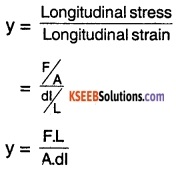where F – Longitudinal force,
L – original length,
dl – change in length,
A – area of cross-section of the wire.

2. Rigidity modulus (η):
It is defined as the ratio of shearing stress to the shearing strain.where F – tangential force,
A – area of the surface,
h – height of the surface,
x – displacement of the surface

3. Bulk modulus (K) :
It is defined as the ratio of bulk stress to the bulk strain. Bulk modulus is given by,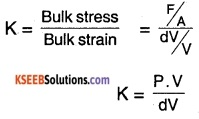where F/A = P – pressure, V is the original volume and dV is the change in volume.

1st PUC Physics Mechanical Properties of Solids Numerical Problems Questions and Answers

Question 1.
What is the percentage increase in length of a wire of diameter 12.5 mm stretched by a weight of 1000 kg ωt ?
[Given Y = 12.5 × 1011 dyne/sq.cm]
Area = $$\frac{\pi d^{2}}{4}=\frac{\pi(1.25)^{2}}{4} \mathrm{cm}^{2}$$
F = 1000 kg ωt
= 1000 × 103 × 980
F = 9.8 × 108 dyne
We know that,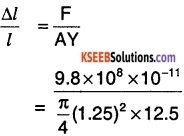$$=\frac{9.8 \times 4 \times 10^{-3}}{\pi \times(12.5)(1.25)^{2}}$$
= 0.638 × 10-3
Percentage increase = 0.064%.

Question 2.
A cube of side 3 cm Is subjected to shearing force. The top of cube is sheared through 0.012 cm with respect to bottom face. If cube is made of a material of shear modulus 2.1 × 1010Nm-2 then find

1. Shearing strain
2. Shearing stress
3. Force applied.

1. Shearing strain
$$=\frac{\Delta l}{l}=\frac{0.012 \mathrm{cm}}{3 \mathrm{cm}}$$
= 0.004

2. Shearing stress
= Shearing strain × Shear modulus
Shearing stress = 0.004 × 2.1 × 1010Nm-1
= 8.4 × 107Nm-2

3. Shearing stress = $$\frac{\text { S.Force }}{\text { Area }}$$
Shearing force = Shearing stress × Area
= 8.4 × 107 × (3 × 10-1)2
= 7.56 × 104 N
= 75.6 KN.

Question 3.
A composite wire of materials A and B are of lengths 3.2 m and 2.4 m, and areas 1cm2 and 1cm2 respectively. If the extension observed is 1.2 mm. Find the force ‘F’. Given YA = 1.6 × 1011 Nm-2 and YB = 2.4 × 101 Nm-2We know that Y = $$\frac{F}{A} \times \frac{l}{\Delta L}$$
Let the individual extension be ∆lA and ∆lB
∆LA = $$F\left(\frac{L_{A}}{A_{A} Y_{A}}\right)$$; ∆LB = $$F\left(\frac{L_{B}}{A_{B} Y_{B}}\right)$$
Given ∆LA + ∆LB = 1.2 mn= 1.2 × 10-4
F(3 × 10-7) = 1.2 × 10-4
F = 400 N

Question 4.
A cube of 5 cm has its upper face displaced by 0.2 cm by a force of 8 N. Find Shear Modulus, Stress and Strain.
Shear stress = $$\frac{F}{A}=\frac{8 N}{25 \times 10^{-4} m^{2}}$$
= 3200 Nm-2
Shear Strain = $$\frac{\Delta l}{l}$$
But ∆l = 0.2 cm
l = 5 cm
Shear strain = $$\frac{0.2 \mathrm{cm}}{5 \mathrm{cm}}$$
= 0.04
Shear Modulus = $$\frac{\text { Shear Stress }}{\text { Shear Strain }}$$
$$=\frac{3200}{0.04}$$
= 80 × 103Nm-2 or 80 kPa.Question 5.
A rubber string 10 m long is suspended from a rigid support at one end. Calculate extension of the string due to its own weight. The density of rubber is 1.5 × 103 kg/m3 and Yrubber = 5 × 106Nm-2.
The weight at any point x from below is, $$\frac{\mathrm{M}}{10}$$ (10 – x) where M is mass of string.
So, Force at any point dx is
F = $$\frac{\mathrm{Mg}}{l}$$ (l – x)dx
We know that,Net extension =So, Net extension of the rubber due to its own weight = $$\frac{1.5 \times 10^{3} \times 10 \times 100}{2 \times 5 \times 10^{6}}$$
= 0.15 m.

Question 6.
A metal rod is tied vertically. If the breaking stress is 5.4 × 109 Nm-2 and density 5.4 × 103 kg/m3. Find the maximum length of rod that can be held without breaking.
Stress = $$\frac{F}{A}$$
= $$\frac{\mathrm{Mg}}{\mathrm{A}}$$ [∵ $$\frac{M}{A L}$$ = ρ]
= ρgL
If ρgL > Maximum stress that it can sustain than the rod break.
So, ρgL < 5.4 × 109 Nm-2
l< $$\frac{5.4 \times 10^{9}}{5.4 \times 10^{3} \times\left(10 m s^{-1}\right)}$$
l < 105 m
Maximum length of wire is 105 m or 100 km..

Question 7.
Consider a tapered wire as shown. Find the extension if Y is its Young’s modulus.dl = $$\left(\frac{F}{A}\right) \frac{d x}{Y}$$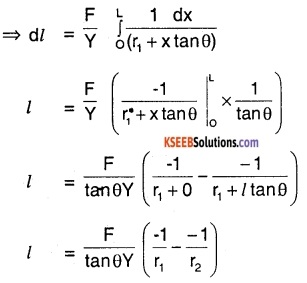Total extension = $$\frac{F\left(r_{2}-r_{1}\right)}{Y \cdot r_{1} \cdot r_{2} \tan \theta}$$
$$=\frac{\mathrm{FL} \tan \theta}{\mathrm{Y} \mathrm{r}_{1}-\mathrm{r}_{2} \tan \theta}$$
Total extension = $$\frac{F \cdot L}{Y r_{1} r_{2}}$$
[∵ tanθ = $$\frac{r_{2}-r_{1}}{L}$$]